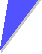# Calculate the Geometry FactorCalculate the geometry factor for diffusion using the formula given forThe fcc latticeThe bcc latticeThe diamond latticeLink to the SolutionLattice and CrystalIonic CrystalsSolution to Exercise 3.1-13.1.1 Diffusion and Point DefectsDiffusion Coefficient and Atomic Mechanisms

© H. Föll (Defects - Script)